## Tuesday, November 14, 2017

### Integer Programming and Least Median of Squares Regression

Least Median Regression  is another attempt to make regression more robust, i.e. less sensitive to outliers.

 \begin{align}\min_{\beta}\>&\operatorname*{median}_i r_i^2 \\ &y-X\beta = r\end{align}

From the summary of :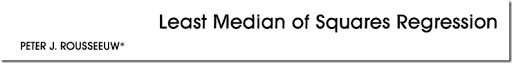Classical least squares regression consists of minimizing the sum of the squared residuals. Many authors have produced more robust versions of this estimator by replacing the square by something else, such as the absolute value. In this article a different approach is introduced in which the sum is replaced by the median of the squared residuals. The resulting estimator can resist the effect of nearly 50% of contamination in the data. In the special case of simple regression, it corresponds to finding the narrowest strip covering half of the observations. Generalizations are possible to multivariate location, orthogonal regression, and hypothesis testing in linear models.

For $$n$$ even, the median is often defined as the average of the two middle observations. For this regression model, usually a slightly simplifying definition is used: the median is the $$h$$-th ordered residual with $$h=\lfloor (n+1)/2\rfloor$$ (here $$\lfloor . \rfloor$$ means rounding down). For technical reasons, sometimes another value is suggested :

 $h=\left\lfloor \frac{n}{2} \right\rfloor + \left\lfloor \frac{p+1}{2}\right\rfloor$

where $$p$$ is the number of coefficients to estimate (number of $$\beta_j$$’s). Some algorithms use $$\lfloor (n+p+1)/2\rfloor$$. The objective function can therefore be described succinctly as:

 $\min\>r^2_{(h)}$

We used here the ordering $$|r|_{(1)}\le |r|_{(2)}\le \dots\le|r|_{(n)}$$. The problem for general $$h$$’s is called least quantile of squares regression or shorter least quantile regression. For $$h=n$$ the model can be reformulated as a Chebyshev regression problem .

##### Intermezzo: Minimizing k-th smallest

Minimizing the $$k$$-th smallest value $$x_{(k)}$$ of a set of variables $$x_i$$, with $$i=1,\dots n$$ is not obvious. Minimizing the largest value, $$x_{(n)}$$ is easy: we can do

 \begin{align}\min\>&z\\&z\ge x_i\end{align}

The trick to minimize $$x_{(k)}$$ is to do:

 \begin{align}\min\>&z\\&z\ge x_i- M \delta_i\\&\sum_i\delta_i = n-k\\&\delta_i \in \{0,1\}\end{align}

In effect we dropped the largest $$n-k$$ values from consideration (in statistical terms this is called “trimming”).  To be precise: we dropped $$n-k$$ values, and because we are minimizing, these will be the largest values automatically. Note that we do not sort the values here: sorting values in a MIP model is very expensive.
##### A quadratic model

The LMS model can now be stated as:

 \begin{align}\min\>&z\\&z\ge r^2_i-M\delta_i\\ &\sum_i \delta_i = n-h\\&r_i = y_i-\sum_j X_{i,j} \beta_j\\&\delta_i \in \{0,1\}\end{align}

This is a MIQCP (Mixed Integer Quadratically Constrained Programming) model. It is convex so we can solve this with solvers like Cplex and Gurobi.

##### An MINLP model

Redefining our $$\delta_i$$ variables, we can formulate a MINLP model:

 \begin{align}\min\>&z\\&z\ge \delta_i \cdot r^2_i\\ &\sum_i \delta_i = h\\&r_i = y_i-\sum_j X_{i,j} \beta_j\\&\delta_i \in \{0,1\}\end{align}

This model is very compact but requires an MINLP solver. Finding globally optimal solutions for this problem is not very easy.

##### Absolute values

Minimizing the median of the squared errors is the same as minimizing the median of the absolute values of the errors. This is because the ordering of squared errors is the same as the ordering of the absolute values. Hence the objective of our problem can be stated as:

 $\min\>|r|_{(h)}$

i.e., minimize the $$h$$-th smallest absolute value.

Now we know how to minimize the $$h$$-th smallest value, the only hurdle left is combining this with an absolute value formulation. We follow here the sparse bounding formulation from [3,4].

 \begin{align}\min\>&z\\&-z - M\delta_i \le r_i \le z + M\delta_i\\&\sum_i \delta_i = n-h\\&r_i = y_i-\sum_j X_{i,j} \beta_j\\&\delta_i \in \{0,1\}\end{align}
It is not so easy to find good values for these big-$$M$$’s (see  for an attempt). One way around this is to use indicator constraints:
 \begin{align}\min\>&z\\&\delta_i=1 \Rightarrow \> –z \le r_i \le z\\&\sum_i \delta_i = h\\&r_i = y_i-\sum_j X_{i,j} \beta_j\\&\delta_i \in \{0,1\} \end{align}

Note that we have flipped the meaning of $$\delta_i$$. If your solver does not allow for indicator constraints, we can also achieve the same thing by SOS1 sets:

 \begin{align}\min\>&z\\&-z-s_i\le r_i \le z + s_i\\&s_i\cdot \delta_i = 0\\&\sum_i \delta_i = h\\&r_i = y_i-\sum_j X_{i,j} \beta_j\\&\delta_i \in \{0,1\}\end{align}

where $$s_i$$ are additional slack variables. They can be free or non-negative variables. The complementarity condition $$s_i\cdot \delta_i = 0$$ can be implemented by forming $$n$$ SOS1 sets with two members: $$(\delta_i,s_i)$$. Actually some solvers may reformulate indicator constraints into SOS1 sets when there are no good bounds (in which case a direct translation to a big-M binary variable reformulation is not possible).

A similar but more complicated approach using variable splitting has been proposed by :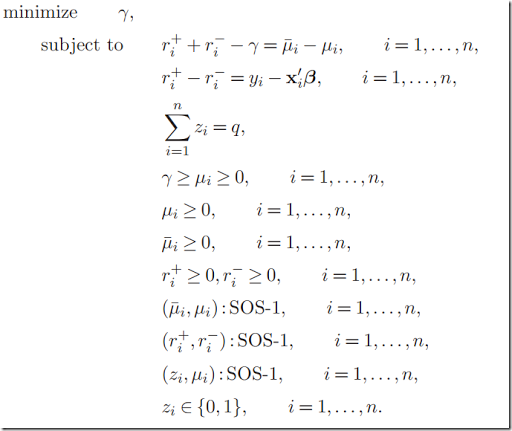Besides being unnecessarily complex, I believe this formulation is not correct. An obvious optimal solution for this model would be $$\gamma=0$$ and $$\mu_i=0$$ which is not a reasonable solution for our original problem. One way to repair this model is by saying the pairs $$(z_i,\bar{\mu}_i)$$ form SOS1 sets instead of $$(z_i,\mu_i)$$. I assume this is what the authors meant. This change makes the model correct, but not any simpler.

If we really want to use a variable splitting approach with SOS1 sets, I propose a much simpler model:

 \begin{align}\min\>&z\\&z \ge r_i^+ + r_i^- - s_i\\&r_i^+ - r_i^- = y_i – \sum_j X_{i,j}\beta_j\\&s_i\cdot \delta_i = 0\\&\sum_i \delta_i = h\\&\delta_i \in \{0,1\}\\&r_i^+, r_i^-, s_i \ge 0\end{align}

The variable splitting in this model is not immediately interpretable: we only guarantee $$|r_i|=r_i^+ + r_i^-$$ for the case where $$s_i=0$$ and $$z \ge r_i^+ + r_i^-$$ is binding.

##### Example

In [6,7] a dramatic example is shown on a small data set.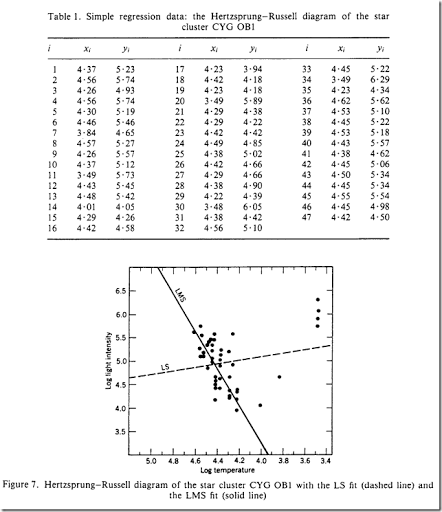Another interesting plot is to compare the ordered absolute values of the residuals: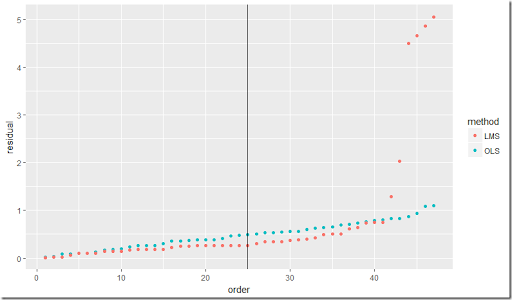Here we plotted $$|r|_{(i)}$$. The model was solved with $$h=25$$ (this is where the vertical line is drawn). As you can see, LMS (Least Median of Squares) tries to keep the absolute value of the $$h$$-th residual as small as possible, and does not care about what happens afterward. We can see the residual increase in magnitude after this point. OLS (Ordinary Least Squares) will try to minimize all squared residuals and thus is heavily influenced by the bigger ones at the end.

##### Some timings

The “k out of n” constraint is always difficult for MIP solvers. We see that also here using Cplex: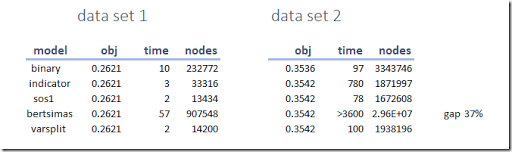Our fixed version of the model from  is doing somewhat poorly: it has too many discrete structures. (In  a really good approximation is found and then passed to the MIP using MIPSTART).

Data set 2 has a few interesting results. The binary variable approach with big-M’s leads to a better than optimal solution due to “trickle flow”. One of the binary variables has value: 3.5193967E-6 which is within the integer feasibility tolerance but is large enough to cause the objective to improve a bit. The Bertsimas model found the optimal solution within an hour but we were not able to prove global optimality (it still has a large relative gap).

##### A note on minimizing the true median

Going back to the standard definition of the median, the “true” median is usually defined as the average of the two middle observations in case $$n$$ is even. In the above discussion we use a simpler definition and just minimized the $$h$$-th ordered residual. Here I’ll try to show that it is possible to handle the traditional definition of the median. This requires a little bit of effort however.

The trick to minimize the median in general can look like :

If $$n$$ is odd:

 \begin{align}\min\>&z\\&z\ge x_i-\delta_i M\\&\sum_i \delta_i = \frac{n-1}{2}\\&\delta_i \in \{0,1\}\end{align}

If $$n$$ is even:

 \begin{align}\min\>&\frac{z_1+z_2}{2}\\&z_1\ge x_i-\delta_i M\\&\sum_i \delta_i = \frac{n}{2}\\&z_2\ge x_i-\eta_i M\\&\sum_i \eta_i = \frac{n}{2}-1\\&\delta_i,\eta_i \in \{0,1\}\end{align}

The “$$n$$ is even” case is the new and interesting part. Note that I expect we can improve this “$$n$$ is even” version a little bit by adding the condition $$\delta_i \ge \eta_i$$.

This technique allows us to calculate the minimum median of a variable $$x_i$$. However, if we want to apply this to our Least Median of Squares model we are in big trouble. In our formulations, we used a trick that minimizing $$r^2_{(h)}$$ is the same as minimizing $$|r|_{(h)}$$. This is no longer the case with this “true” median objective for $$n$$ even. We can however use a quadratic formulation:

If $$n$$ is even:

 \begin{align}\min\>&\frac{z_1+z_2}{2}\\&z_1\ge r^2_i-\delta_i M\\&\sum_i \delta_i = \frac{n}{2}\\&z_2\ge r^2_i-\eta_i M\\&\sum_i \eta_i = \frac{n}{2}-1\\&r_i = y_i-\sum_j X_{i,j} \beta_j\\&\delta_i,\eta_i \in \{0,1\}\end{align}

Although we can solve this with standard solvers, it turns out to be quite difficult. The linear models discussed before were much easier to solve.

This regression problem turns out to be interesting from a modeling perspective.

##### References
1. Peter Rousseeuw, Least Median of Squares Regression, Journal of the American Statistical Association, 79 (1984), pp. 871-880.
2. A. Giloni, M. Padberg, Least Trimmed Squares Regression, Least Median Squares Regression, and Mathematical Programming, Mathematical and Computer Modelling 35 (2002), pp. 1043-1060
3. Linear programming and LAD regression, http://yetanothermathprogrammingconsultant.blogspot.com/2017/11/lp-and-lad-regression.html
4. Linear programming and Chebyshev regression, http://yetanothermathprogrammingconsultant.blogspot.com/2017/11/lp-and-chebyshev-regression.html
5. Dimitris Bertsimas and Rahul Mazumder, Least Quantile Regression via Modern Optimization, The Annals of Statistics, 2014, Vol. 42, No. 6, 2494-2525, https://arxiv.org/pdf/1310.8625.pdf.
6. Peter Rousseeuw, Annick Leroy, Robust Regression and Outlier Detection, Wiley, 2003.
7. Peter Rousseeuw, Tutorial to Robust Statistics, Journal of Chemometrics, Vol. 5, pp. 1-20, 1991.
8. Paul Rubin, Minimizing a Median, https://orinanobworld.blogspot.com/2017/09/minimizing-median.html